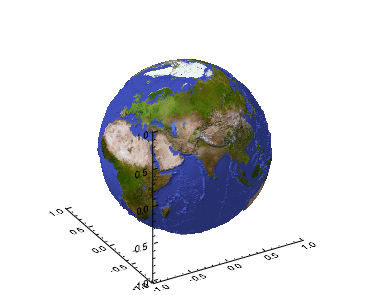The example below shows how to display a digital elevation model (DEM) on an image and display it as a three-dimensional globe.The code shown below creates the graphic shown above. Copy the code below and paste it into a new file in the IDL editor, then compile and run it.

`PRO WORLD_SURF`
` `
`; Read the DEM data file.`
`OPENR, lun, FILEPATH('worldelv.dat', \$`
`  SUBDIR = ['examples', 'data']), /GET_LUN`
`elev = BYTARR(360, 360)`
` `
`; Read the unformatted binary file data into a variable.`
`READU, lun, elev`
` `
`; Deallocate file units associated with GET_LUN.`
`FREE_LUN, lun`
`  elev = SHIFT(elev, 180)`
`  zscale = 0.05`
`  a = 360L`
`  b = 360L`
`  n = a  * b`
`  spherical = MAKE_ARRAY(3, n, /DOUBLE)`
`  FOR i = 0L, a - 1 DO BEGIN`
`    FOR j = 0L, b - 1 DO BEGIN`
`      k = ( i * b ) + j`
`      spherical[0, k] = j * 360.0 / (a - 1)            ; longitude [0.0, 360.0]`
`      spherical[1, k] = i * 180.0 / (b - 1) - 90.0     ; latitude [90.0, -90.0]`
`      spherical[2, k] = 1.0 + zscale * elev[k] / 255.0 ; radius`
`    ENDFOR`
`  ENDFOR`
` `
`; Convert the spherical coordinates to rectangular coordinates.`
`rectangular = CV_COORD(FROM_SPHERE = spherical, /TO_RECT, /DEGREES)`
`z = REFORM( rectangular[2, *], a, b )`
`x = REFORM( rectangular[0, *], a, b )`
`y = REFORM( rectangular[1, *], a, b )`
`; Read the global map file data.`
`im = read_png(FILEPATH('avhrr.png', SUBDIR = ['examples', 'data']), r, g, b)`
` `
`; Create the array for use by the TEXTURE_IMAGE keyword for SURFACE.`
`image = BYTARR(3, 720, 360)`
`IMAGE[0, *, *] = r[im]`
`IMAGE[1, *, *] = g[im]`
`IMAGE[2, *, *] = b[im]`
` `
`; Display the surface.`
`s = SURFACE(z, x, y, TEXTURE_IMAGE = image, LOCATION = [0, 0], aspect_z=1.0)`
` `
`END`